Next: Representation of Angular Momentum Up: Orbital Angular Momentum Previous: Introduction

# Angular Momentum Operators

In classical mechanics, the vector angular momentum, L, of a particle of position vectorand linear momentumis defined as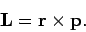(526)

It follows that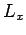(527)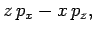(528)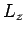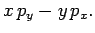(529)

Let us, first of all, consider whether it is possible to use the above expressions as the definitions of the operators corresponding to the components of angular momentum in quantum mechanics, assuming that theand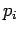(where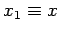,,, etc.) correspond to the appropriate quantum mechanical position and momentum operators. The first point to note is that expressions (527)-(529) are unambiguous with respect to the order of the terms in multiplicative factors, since the various position and momentum operators appearing in them all commute with one another [see Eqs. (483)]. Moreover, given that theand theare Hermitian operators, it is easily seen that the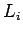are also Hermitian. This is important, since only Hermitian operators can represent physical variables in quantum mechanics (see Sect. 4.6). We, thus, conclude that Eqs. (527)-(529) are plausible definitions for the quantum mechanical operators which represent the components of angular momentum.

Let us now derive the commutation relations for the. For instance,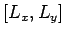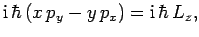(530)

where use has been made of the definitions of the[see Eqs. (527)-(529)], and commutation relations (481)-(483) for theand. There are two similar commutation relations: one for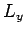and, and one forand. Collecting all of these commutation relations together, we obtain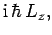(531)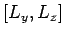(532)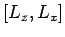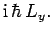(533)

By analogy with classical mechanics, the operator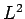, which represents the magnitude squared of the angular momentum vector, is defined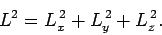(534)

Now, it is easily demonstrated that ifandare two general operators then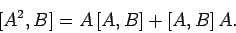(535)

Hence,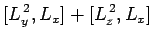(536)

where use has been made of Eqs. (531)-(533). In other words,commutes with. Likewise, it is easily demonstrated thatalso commutes with, and with. Thus,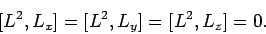(537)

Recall, from Sect. 4.10, that in order for two physical quantities to be (exactly) measured simultaneously, the operators which represent them in quantum mechanics must commute with one another. Hence, the commutation relations (531)-(533) and (537) imply that we can only simultaneously measure the magnitude squared of the angular momentum vector,, together with, at most, one of its Cartesian components. By convention, we shall always choose to measure the-component,.

Finally, it is helpful to define the operators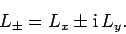(538)

Note that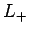and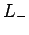are not Hermitian operators, but are the Hermitian conjugates of one another (see Sect. 4.6): i.e.,(539)

Moreover, it is easily seen that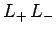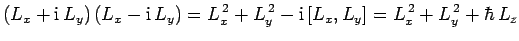(540)

Likewise,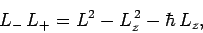(541)

giving(542)

We also have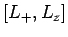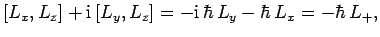(543)

and, similarly,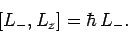(544)Next: Representation of Angular Momentum Up: Orbital Angular Momentum Previous: Introduction
Richard Fitzpatrick 2010-07-20